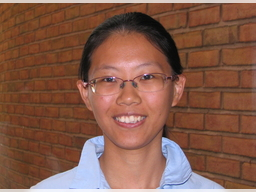← Back to Event List

# Doctoral Dissertation Defense: Mingkai Yu

Location

Mathematics/Psychology : 412

Date & Time

May 5, 2023, 11:00 am1:00 pm

Description

Title:  State and Parameter Estimation in Stochastic Dynamical Systems

Abstract
Stochastic dynamical systems arise in the modeling of intracellular biological processes driven by diffusion and reaction of molecules. While several fluorescence based techniques exist for partial measurements of these systems, it remains a challenge to estimate the full state and parameters of such systems from the partially observed data. In this thesis, we study two problems: inferring the diffusivity from raw Fluorescence Correlation Spectroscopy (FCS) data, and estimating state and parameters in partially observed chemical reaction networks.

Fluorescence intensity could be modeled as a stochastic process governed by the motion of particles. The autocorrelation of the intensity has an analytical form as a function of time lag and diffusion coefficient, hence the diffusion coefficient could be fitted. We present a mathematical derivation of the autocorrelation function. We also derive a formula for the variance of the time average of the autocorrelation function in integral form, and give a closed-form upper bound. We examine several different approaches to fit the diffusivity via Monte Carlo simulations.  Furthermore, we analyze the sensitivity of the diffusivity D obtained via the process of nonlinear least squares fit.

We also study chemical reaction networks modeled by a discrete-state continuous-time Markov process, where the state vector represents the copy number of the species. We consider two scenarios, one in which exact observations of some species are made continuously in time over a window and the other in which observations are made in snapshots of time.  For the continuous in time observation problem, we derive equations for the conditional probability distribution of the unobserved states.  We also provide a novel particle filter to compute the conditional probability distribution of the unobserved species. We also adapt our algorithm for the estimation of parameters and for a past state value based on observations up to a future time.

For the filtering of stochastic reaction networks when some states are observed noiselessly in snapshots of time, we propose a targeting algorithm that ensures the filter process reaches the target state. We present a rigorous proof of our algorithm, discuss the choices we could make within the implementation of the algorithm, and use numerical examples to illustrate our algorithm.

Tags: